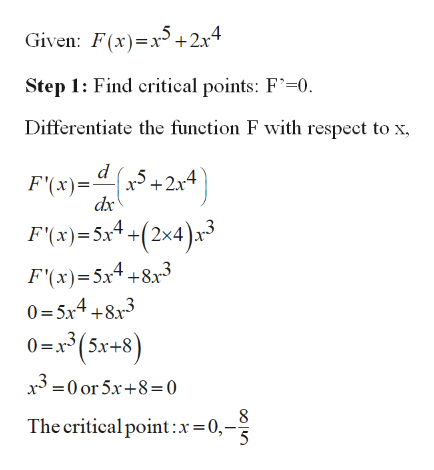# Consider the following function: F(x) = x^5 + 2x^4Identify the following:d. Intervals where the function is increasing/decreasinge. Local extrema, give the location and value for any local maxima/minimaf. Concvity of the function and Points of Inflection (if they exist)

Question
8 views

Consider the following function:

F(x) = x^5 + 2x^4

Identify the following:

d. Intervals where the function is increasing/decreasing

e. Local extrema, give the location and value for any local maxima/minima

f. Concvity of the function and Points of Inflection (if they exist)

check_circle

Step 1

Part (d):

Increasing and decreasing:help_outlineImage TranscriptioncloseGiven: F(x)x5+2x4 Step 1: Find critical points: F-0. Differentiate the function F with respect to x X5+2x4 F'(x) dx F'(x)5x4 +(2x4)x F'(x)-5x4+83 0= 5x4 +8x3 0=x3(5x+8 x30 or 5x+8=0 The critical point :x=0,- 5 fullscreen
Step 2

Step 2: Test points to find interval increasing or decreasing.

If F’(x) >0, the function is increasing.

If F’(x) <0, the function is increasing.

Step 3

Part (e):

The sign changes + to – in F’(c...

### Want to see the full answer?

See Solution

#### Want to see this answer and more?

Solutions are written by subject experts who are available 24/7. Questions are typically answered within 1 hour.*

See Solution
*Response times may vary by subject and question.
Tagged in

### Functions